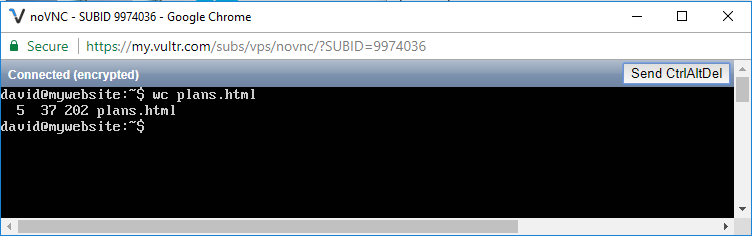﻿ How to Count the Number of Lines, Words, and Characters of a File in Linux ﻿# How to Count the Number of Lines, Words, and Characters of a File in LinuxIn this article, we show how to count the number of lines, words, and characters of a file in linux.

So Linux is able to analyze files and give back details of a file.

One of the things it can do in file analysis is give back the number of lines in a file, the number of words in a file, and the number of characters in a file.

So we can get this information in linux using the wc command.

The wc command gives us back 3 values.

The first value is the number of lines in the file.

The second value is the number of words in the file.

The third value is the number of characters in the file.

So I'm going to create a file and name it, plans.html

I placed this file in the current working directory.

In the following code below, we get the number of lines, words, and characters of the file in linux.

So we specify wc plans.html.

This shows us the number of lines, words, and characters in the plans.html file.

This is shown in the following output below.5 is the number of lines, 37 is the number of words, and 202 is the number of characters in the file.

I believe that linux starts line count at an index of 0, because in the plans.html file, there are actually 6 lines, not 5.

If you want to get the individual values (lines, words, or counts) separately rather than all together, you can do so.

To get the number of lines in a file, we use the following code below.

This gets the number of lines in the file in linux.

To get the number of words in a file, we use the following code below.

This gets the number of words in the file in linux.

To get the number of characters in a file, we use the following code below.

This gets the number of characters in the file in linux.

All of these are shown in the output below.So you can see that we've obtained the number of lines, words, and characters separately of the plans.html in linux.

Related Resources

How to Randomly Select From or Shuffle a List in Python